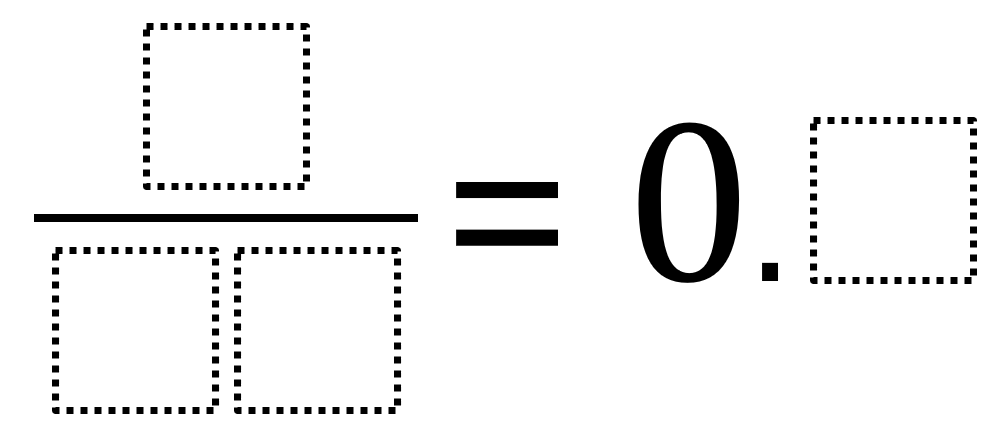# Converting a Fraction to a Decimal

Directions: Using the digits 1 to 9, at most one time each, place a digit in each box to make a true statement.### Hint

How does choosing the digits for the denominator affect the decimal value?
How might choosing the digit for the decimal make finding the digits for the fraction easier?

Number of Unique Solutions: 9
1:   3 / 15 = 0.2
2:   6 / 12 = 0.5
3:   6 / 15 = 0.4
4:   7 / 14 = 0.5
5:   7 / 35 = 0.2
6:   8 / 16 = 0.5
7:   9 / 15 = 0.6
8:   9 / 18 = 0.5
9:   9 / 45 = 0.2

Source: Owen Kaplinsky

## Similar Triangles And Slope

Directions: The three triangles on the line are similar. Using the digits 0 to 9 …

1.•2.nice problem! the answers I get are:

0.1 – not possible
0.2 – 3/15 or 7/35
0.3 – not possible
0.4 – 6/15
0.5 – 6/12, 7/14, 8/16, 9/18
0.6 – 9/15
0.7 – not possible
0.8 – not possible
0.9 – not possible

•Some of my students also found 9/45 = 0.2 🙂

•Why not 6/20 = 0.3?

•3.Great problem!

4.5.1/10= 0.1

•You can only use each digit once, so you can’t use three ones

6.I think it’s 1/10 = 0.1

7.” how does choosing the digits for the denominator affect the decimal value.” The denominator has to be able to be divided by ten. The fraction in it lowest form has to be able to be converted into its lowest tenths. ” how might choosing the digit for the decimal make finding the digit for the fraction easier”.The decimal amount can just get put over a tenth.

•Thanks for your contribution! I am working on adding more tasks like this into my weekly/daily routines and people like you adding on to these tasks makes it so much easier!!

8.9.10.